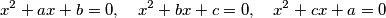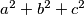MEMO 2009 ekipno problem 2

Kvaliteta:
Avg: 2,0
Težina:
Avg: 6,0
Let$a$,$b$,$c$ be real numbers such that for every two of the equationsthere is exactly one real number satisfying both of them. Determine all possible values of$a^2+b^2+c^2$.
Izvor: Srednjoeuropska matematička olimpijada 2009, ekipno natjecanje, problem 2